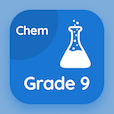Engineering Online Courses

Engineering Physics Quizzes

Engineering Physics Quiz PDF - Complete

# Melting Points Multiple Choice Questions p. 74

Study Melting Points multiple choice questions and answers, melting points quiz answers PDF 74 to study Engineering Physics course online. Properties of Common Elements MCQ trivia questions, Melting Points Multiple Choice Questions (MCQ) for online college degrees. "Melting Points MCQ" PDF eBook: gravitation near earth surface, diffraction by a single slit, work and rotational kinetic energy, molar specific heat of ideal gases, melting points test prep for engineering associate's degree online.

"1287°C is melting point of" MCQ PDF: astarine, beryllium, arsenic, and dubnium for grad school interview questions. Learn properties of common elements questions and answers to improve problem solving skills for college entrance examination.

## Melting Points Questions and Answers MCQs

MCQ: 1287°C is melting point of

Beryllium
Astarine
Arsenic
Dubnium

MCQ: Molar specific speed of Nitrogen gas is

202.7 J/mol.K
20.7 J/mol.K
12.6 J/mol.K
125 J/mol.K

MCQ: Product of torque and angular velocity is equals to

power
kinetic energy
rotational inertia
angular acceleration

MCQ: If wave's first side diffraction maximum is 15° and is coinciding with first minimum of red light, then wavelength of light is

430 nm
120 nm
350 nm
1200 nm

MCQ: If M is earth's mass, r is the distance between them and G is gravitational constant then by Newton's law of gravitation gravitational acceleration is equals to

GM/r
rG/M
GMr
GM/r2

### More Quizzes from Engineering Physics Course